# ₦airaland Forum

Welcome, Guest: Join Nairaland / LOGIN! / Trending / Recent / New
Stats: 2,298,348 members, 5,051,398 topics. Date: Monday, 22 July 2019 at 03:02 PM

## This Is Treasure Hunt (mr Shape) - Education - Nairaland

 This Is Treasure Hunt (mr Shape) by Mrshape: 11:49am On May 04 Find the square , if you can find it, them find its area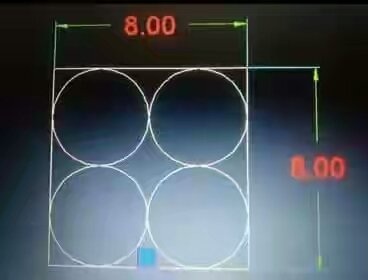Re: This Is Treasure Hunt (mr Shape) by Mrshape: 12:47pm On May 04 Anyone? Re: This Is Treasure Hunt (mr Shape) by femi4: 1:36pm On May 04 (6-X)²+4² = 4² +(4+X)²36-12X+ X²= 16 + 8X + X²20x = 20X = 1Area of the square = 1² = 1unit² Re: This Is Treasure Hunt (mr Shape) by Martinez39: 5:20pm On May 04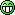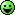A = (24 - 8√5) ÷ 5 ≈ 1.22229124 unit².Cc. Mrshape Re: This Is Treasure Hunt (mr Shape) by Mrshape: 6:45pm On May 04 Show your workings Re: This Is Treasure Hunt (mr Shape) by Mrshape: 7:45am On May 05 femi4:(6-X)²+4² = 4² +(4+X)²36-12X+ X²= 16 + 8X + X²20x = 20X = 1Area of the square = 1² = 1unit²Try again sir Re: This Is Treasure Hunt (mr Shape) by Mrshape: 7:45am On May 05 Martinez39:A = (24 - 8√5) ÷ 5 ≈ 1.22229124 unit².Cc. Mrshape Try again sir Re: This Is Treasure Hunt (mr Shape) by Mrshape: 7:54am On May 05 Should I drop workings? Re: This Is Treasure Hunt (mr Shape) by Darivie04(m): 8:27am On May 05 Mrshape:Should I drop workings?Yes Re: This Is Treasure Hunt (mr Shape) by femi4: 8:46am On May 05 [quote author=Mrshape post=78115557][/quote]This should do itA rectangle is formed at the centre by merging the two squares.So if x is the length of the squareL of the rectangle = 2xb of the rectangle = length of the square = xDiagonal = x√5Now4² + 4² = (4+ x√5)²32 = (4+x√5)²4+x√5 = 4√2x = (4√2 - 4)/√5Area of the square = x² = (4√2 - 4 )²/5 = 0.5490unit²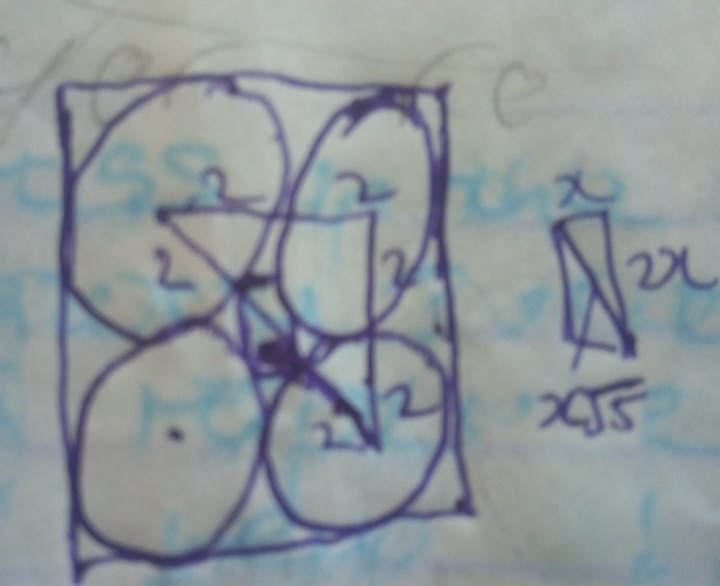Re: This Is Treasure Hunt (mr Shape) by RolD: 9:15am On May 05 https://www.nairaland.com/5169853/unilag-student-adedoyin-wins-national Re: This Is Treasure Hunt (mr Shape) by Mrshape: 10:13am On May 05 femi4:This should do itA rectangle is formed at the centre by merging the two squares.So if x is the length of the squareL of the rectangle = 2xb of the rectangle = length of the square = xDiagonal = x√5Now4² + 4² = (4+ x√5)²32 = (4+x√5)²4+x√5 = 4√2x = (4√2 - 4)/√5Area of the square = x² = (4√2 - 4 )²/5 = 0.5490unit²No sir, I will post solution soon Re: This Is Treasure Hunt (mr Shape) by Mrshape: 10:27am On May 05 Solution.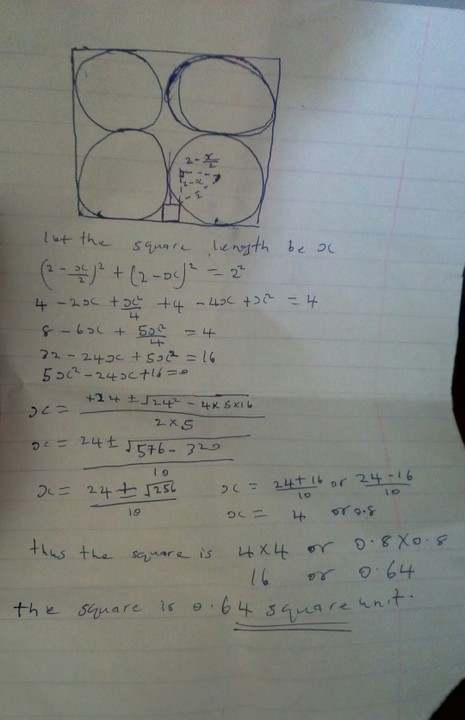Re: This Is Treasure Hunt (mr Shape) by Mrshape: 10:32am On May 05 If you have any objection or questions regarding my solution feel free to ask. Re: This Is Treasure Hunt (mr Shape) by Darivie04(m): 11:34am On May 05 Mrshape:Solution.Can you explain some of the intuition and reasoning behind the solution in words? Re: This Is Treasure Hunt (mr Shape) by Mrshape: 11:39am On May 05 Darivie04:Can you explain some of the intuition and reasoning behind the solution in words?Ok sir I will Re: This Is Treasure Hunt (mr Shape) by Mrshape: 11:56am On May 05 Darivie04:Can you explain some of the intuition and reasoning behind the solution in words?Divide the Shape into two parts by drawing a symmetrical line as shown in the diagram.Since the square is between two circles it is obvious the line will divide the square into a rectangle of top lenght 1/2(lenght of square and the other side of the rectangle will be also equal to the square lenght because it was not dividedNext form a right angled triangle by projecting a vertical line from the vartice of the rectangle to interseect at right angle with an horizontal line draw from the center of the circle.Note the vertical line will (radius (2)-lenght of square)The horizontal line will be (radius(2) -1/2 lenght of the square)And the hypotenuse is =radius (2)Since the vertical and horizontal line must intersect at right angle(2-0.5x)^2+(2-x)^2=2^2.The rest are algebra...... Re: This Is Treasure Hunt (mr Shape) by Mrshape: 12:00pm On May 05 femi4:This should do itA rectangle is formed at the centre by merging the two squares.So if x is the length of the squareL of the rectangle = 2xb of the rectangle = length of the square = xDiagonal = x√5Now4² + 4² = (4+ x√5)²32 = (4+x√5)²4+x√5 = 4√2x = (4√2 - 4)/√5Area of the square = x² = (4√2 - 4 )²/5 = 0.5490unit²From your diagram the diagonal of the right angle must not always pass through both centers of the circles the vartices are touching,That's were you got it wrong sir. Re: This Is Treasure Hunt (mr Shape) by femi4: 1:23pm On May 05 Mrshape:If you have any objection or questions regarding my solution feel free to ask.No objection, I tried that route and got some negative values. 1 Like Re: This Is Treasure Hunt (mr Shape) by Mrshape: 1:37pm On May 05 femi4:No objection, I tried that route and got some negative values. You are welcome sir.